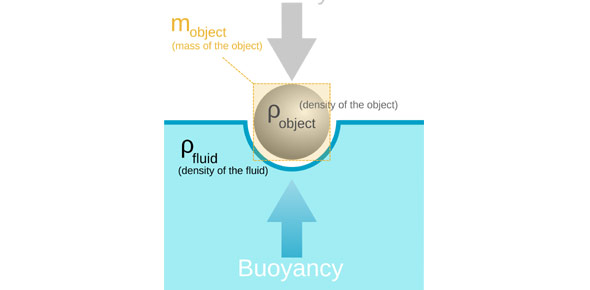# A Simple Multiple Choice Quiz On Floating And Sinking

Approved & Edited by ProProfs Editorial Team
The editorial team at ProProfs Quizzes consists of a select group of subject experts, trivia writers, and quiz masters who have authored over 10,000 quizzes taken by more than 100 million users. This team includes our in-house seasoned quiz moderators and subject matter experts. Our editorial experts, spread across the world, are rigorously trained using our comprehensive guidelines to ensure that you receive the highest quality quizzes.
| By Andreav
A
Andreav
Community Contributor
Quizzes Created: 1 | Total Attempts: 1,776
Questions: 8 | Attempts: 1,792Settings• 1.

### What is Density

• A.

Height of an object

• B.

An objects mass per unit of volume

B. An objects mass per unit of volume
Explanation
Density is a measure of how much mass is contained within a given volume of an object. It is calculated by dividing the mass of the object by its volume. Therefore, the correct answer is "an object's mass per unit of volume" because it accurately describes the concept of density.

Rate this question:

• 2.

• 3.

### An object will float on the surface of a fluid when it is ____________________than the fluid. (answer in two words)

less dense
Explanation
When an object is less dense than the fluid it is placed in, it will float on the surface of the fluid. This is because the buoyant force exerted on the object by the fluid is greater than the gravitational force pulling the object downward. As a result, the object displaces a volume of fluid equal to its own volume, causing it to float and remain on the surface of the fluid.

Rate this question:

• 4.

### What can changing the Density explain?

• A.

Why an object can float or sink

• B.

The reason of how much bouyancy is in an object

• C.

The amount of pressure

A. Why an object can float or sink
Explanation
Changing the density of an object can explain why it can float or sink. Density is the measure of how much mass is contained in a given volume. If the density of an object is less than the density of the fluid it is placed in, it will float because it is less dense and displaces an amount of fluid equal to its weight. On the other hand, if the density of the object is greater than the density of the fluid, it will sink because it is denser and cannot displace enough fluid to support its weight.

Rate this question:

• 5.

• 6.

### What is bouyant force?

• A.

Water and other fluids exert an upward force

• B.

The opposite of gravity and weight

A. Water and other fluids exert an upward force
Explanation
The bouyant force is the upward force exerted by water and other fluids on an object submerged or floating in it. This force is the opposite of gravity and weight, causing objects to feel lighter or even float in water. It is a result of the pressure difference between the top and bottom of the object, with the higher pressure at the bottom pushing it upward. This force is what allows objects to float and is the reason why we feel lighter when submerged in water.

Rate this question:

• 7.

### __________States that the bouyant force acting on a submerged object is equal to weight of the volume of a displaced by the object

Archimedes principle
Explanation
Archimedes' principle states that the buoyant force acting on a submerged object is equal to the weight of the volume of fluid displaced by the object. This principle explains why objects float or sink in a fluid. When an object is submerged in a fluid, it displaces a certain volume of fluid. The buoyant force exerted on the object is equal to the weight of the fluid displaced, which can either be greater or less than the weight of the object itself. If the buoyant force is greater, the object will float; if it is less, the object will sink. This principle is essential in understanding the behavior of objects in fluids.

Rate this question:

• 8.

### How does a helium balloon float in the air

Related TopicsBack to top